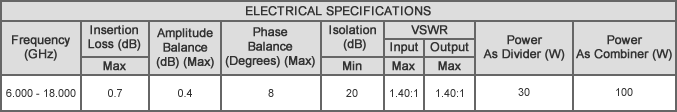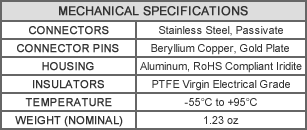# P3S-12.000, SMA-Female, 6.0-18.0 GHz

Original price - Original price
Original price
-
Current price
SKU P3S-12.000

Description:
MECA's 3-way, P3S-12.000, Tee SMA power divider/combiner with power divider of 30 watts, power combiner of 100 watts frequency of 6.0-18.0 GHz.Notes:
1. All units rated for maximum input power as a divider or balanced combiner with load
VSWR of 1.20:1 or better. To calculate the combining rated power the following formula is used:
(rated input power of divider * 5%) / "N" # of input channels = max input at each port for combining.
2. All output/power combiner ports are in-phase (0° difference).
3. 50 ohm nominal impedance standard.
4. Operating temperature from -55°C to +95°C.